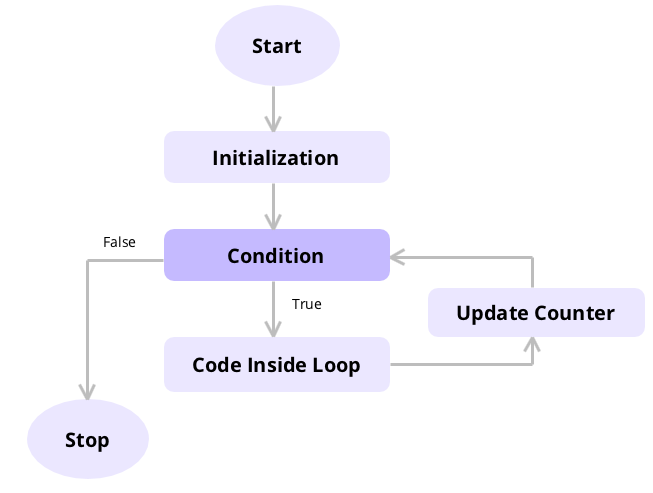Code has been added to clipboard!

# For Loop C++: Everything Covered

Published Sep 3, 2019
Updated Jan 20, 2020

#### Web Development Course:

TL;DR – The loop function executes a block of statements repeatedly. The `for` loop function is used when you need to execute code multiple times.

## What is a C++ for loop?

Unlike the other two types of loops, the `for` loop evaluates the condition before executing the code. As this function uses a boolean condition, the loop will run if the condition is fulfilled or returns a TRUE value.

The condition is then re-checked to see whether or not it’s still met. The loop only stops if the condition is no longer met or returns a FALSE value.## C++ for loop Syntax

Below is the basic syntax of the `for` loop statement in C++.

Example
``````for (initializationStatement; testExpression; updateStatement) {
// code
}``````

The `initializationStatement` is executed only once as its function is to declare the variable’s value. The `testExpression` is the condition that is used to evaluate the variable. If the `testExpression` returns a TRUE value, then the `updateStatement` will be executed to produce a new value for the variable.

## Creating C++ loops

In the code example below, the variable a has an initial value of 10. The value is checked on whether or not it’s less than 20. If it’s true, then the value will be increased by 1 and printed after the text `value of a:`. The loop stops when the variable a has a value equal to 20 and over.

Example
``````#include <iostream>

using namespace std;

int main() {
// for loop execution
for (int a = 10; a < 20; a = a + 1) {
cout << "value of a: " << a << endl;
}
}``````

When the syntax shown above is executed, this will be the output:

Example
``````value of a: 10
value of a: 11
value of a: 12
value of a: 13
value of a: 14
value of a: 15
value of a: 16
value of a: 17
value of a: 18
value of a: 19``````

While creating loops, an infinite loop can happen if you set the condition `never` to return FALSE value. As a result, the loop will never stop as the condition always returns a TRUE value.

Example
``````using namespace std;

int main() {
int temp = 0;
while (temp != 1) {
/*
the code added here will loop forever
*/
}
}``````

To avoid creating an infinite loop, be sure to pick a condition that can serve as the maximum threshold of said value.

## Display array elements using for loops

You can also use the for loop to display elements within an array. It works similarly to using a normal variable.

Starting with a value of 0, the loop will check whether or not the value of the element is less than 5. If it’s true, the element will be printed, and the loop will start over. The loop stops once all the elements have been assessed.

Example
``````#include <iostream>

using namespace std;

int main() {
int arr[] = {
21,
9,
56,
99,
202
};
/* Value of variable i is set to 0 as the array index starts with 0
* so the first element of array starts with zero index.
*/
for (int i = 0; i < 5; i++) {
cout << arr[i] << endl;
}
}``````

## The for loop in C++: useful tips

• As this type of loop checks the condition prior to executing the code, it’s best to use a condition that matches with the variable’s initial value.
• Be sure to use a condition that can return a FALSE value at some point to avoid creating an infinite loop.